1. 滑动窗口
1. 76-最小覆盖子串

# 滑动窗口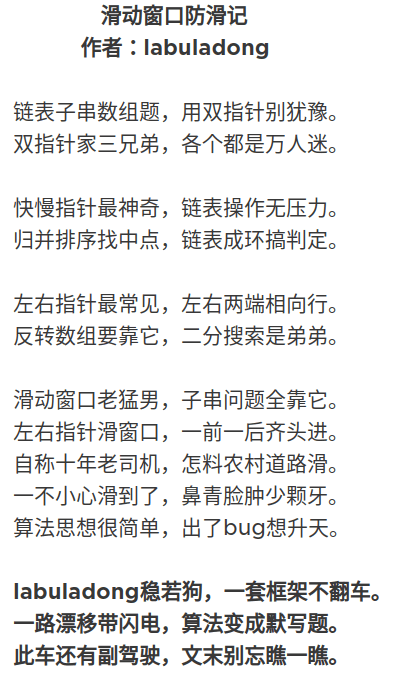``````int left = 0, right = 0;

while (right < s.size()) {
// 增大窗口
right++;

while (window needs shrink) {
// 缩小窗口
window.remove(s[left]);
left++;
}
}
``````

• 比如说如何向窗口中添加新元素，如何缩小窗口，在窗口滑动的哪个阶段更新结果。

• 即便你明白了这些细节，也容易出 bug，找 bug 还不知道怎么找，真的挺让人心烦的。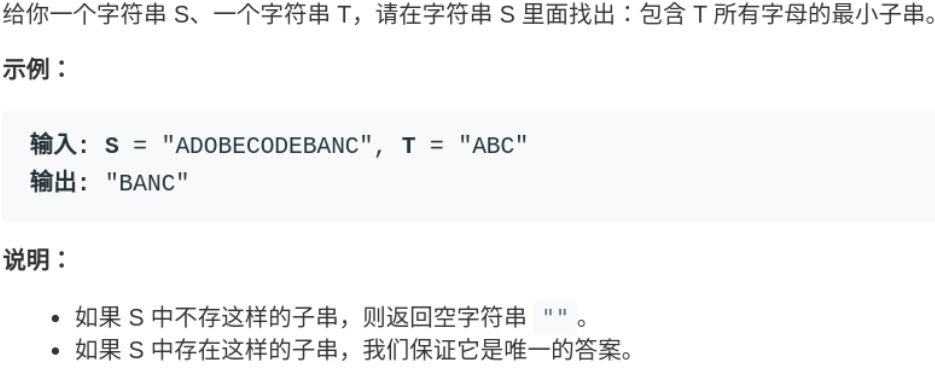``````/* 滑动窗口算法框架 */
void slidingWindow(string s, string t) {
Map<Character, Integer> window = new HashMap<>(16);
Map<Character, Integer> need = new HashMap<>(16);
for(char c : t.toCharArray()) {
// getOrDefault: 如果 need 中没有c就返回0
need.put(c, need.getOrDefault(c, 0)+1);
}

int left = 0, right = 0;
int valid = 0;
while (right < s.length()) {
// c 是将移入窗口的字符
char c = s.charAt(right);
// 右移窗口
right++;
// 进行窗口内数据的一系列更新
...

/*** debug 输出的位置 ***/
printf("window: [%d, %d)\n", left, right);
/********************/

// 判断左侧窗口是否要收缩
while (window needs shrink) {
// d 是将移出窗口的字符
char d = s.charAt(left);
// 左移窗口
left++;
// 进行窗口内数据的一系列更新
...
}
}
}
``````

## 76-最小覆盖子串

https://leetcode-cn.com/problems/minimum-window-substring/

• 对于 t 中重复字符，我们寻找的子字符串中该字符数量必须不少于 t 中该字符数量。
• 如果 s 中存在这样的子串，我们保证它是唯一的答案。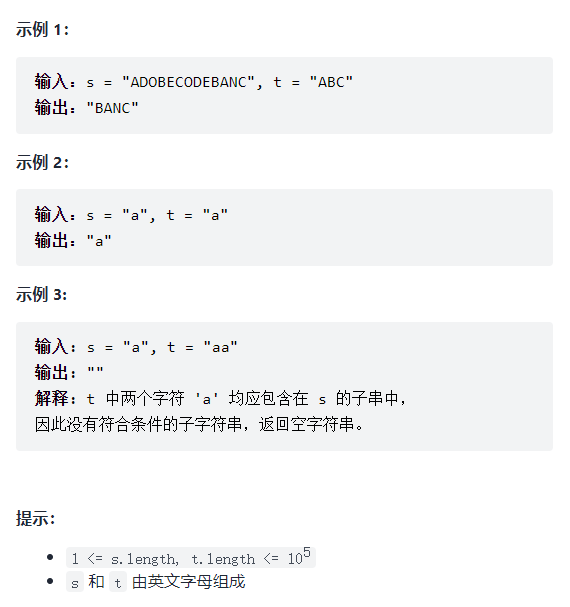``````for (int i = 0; i < s.size(); i++)
for (int j = i + 1; j < s.size(); j++)
if s[i:j] 包含 t 的所有字母:
更新答案
``````

1、我们在字符串 `S` 中使用双指针中的左右指针技巧，初始化 `left = right = 0`，把索引左闭右开区间 `[left, right)` 称为一个「窗口」。

2、我们先不断地增加 `right` 指针扩大窗口 `[left, right)`，直到窗口中的字符串符合要求（包含了 `T` 中的所有字符）。

3、此时，我们停止增加 `right`，转而不断增加 `left` 指针缩小窗口 `[left, right)`，直到窗口中的字符串不再符合要求（不包含 `T` 中的所有字符了）。同时，每次增加 `left`，我们都要更新一轮结果。

4、重复第 2 和第 3 步，直到 `right` 到达字符串 `S` 的尽头。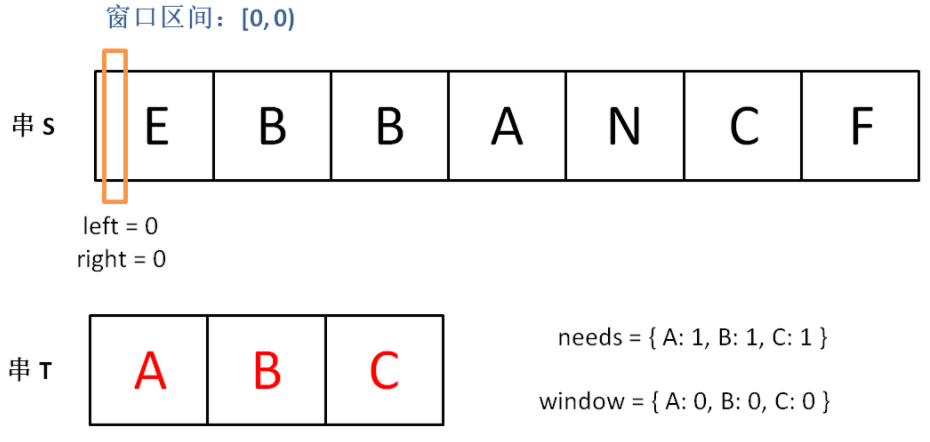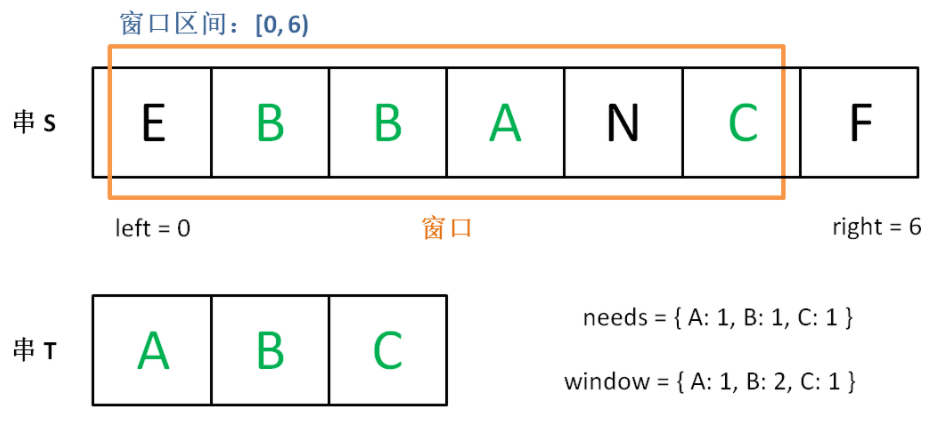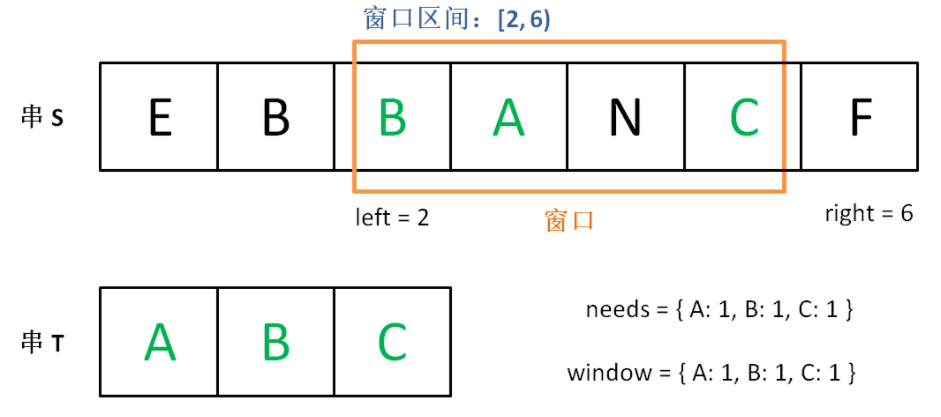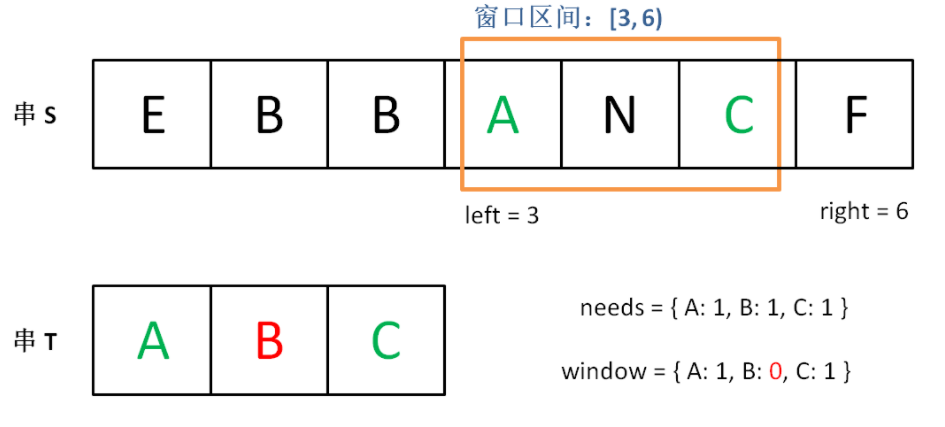``````Map<Character, Integer> window = new HashMap<>(16);
Map<Character, Integer> need = new HashMap<>(16);
for(char c : t.toCharArray()) {
need.put(c, need.getOrDefault(c, 0)+1);
}
``````

``````int left = 0, right = 0;
int valid = 0;
while (right < s.size()) {
// 开始滑动
}
``````

1、当移动 `right` 扩大窗口，即加入字符时，应该更新哪些数据？

2、什么条件下，窗口应该暂停扩大，开始移动 `left` 缩小窗口？

3、当移动 `left` 缩小窗口，即移出字符时，应该更新哪些数据？

4、我们要的结果应该在扩大窗口时还是缩小窗口时进行更新？

`valid` 满足 `need` 时应该收缩窗口；

``````package com.slidingwindow;

import java.util.HashMap;
import java.util.Map;
import java.util.Scanner;

public class minWindow_76 {
public static void main(String[] args) {
// "ABC"
// "BANC"
String res = minWindow(s, t);
System.out.println(res);
}

/**
* 返回 s 中 涵盖 t 所有字符的最小子串
* ABC
* */
public static String minWindow(String s, String t) {
Map<Character, Integer> window = new HashMap<>(16);
Map<Character, Integer> need = new HashMap<>(16);
for(char c : t.toCharArray()) {
need.put(c, need.getOrDefault(c, 0)+1);
}

int left = 0, right = 0;
int valid = 0;
// 记录最小覆盖子串的起始索引及长度
int start = 0, len = Integer.MAX_VALUE;
while (right < s.length()) {
// c 是将移入窗口的字符
char c = s.charAt(right);
// 右移窗口
right++;
// 进行窗口内数据的一系列更新
if (need.containsKey(c)) {
window.put(c, window.getOrDefault(c, 0)+1);
if (window.get(c).equals(need.get(c))) {
valid++;
}
}

// 判断左侧窗口是否要收缩
while (need.size() == valid) {
// 在这里更新最小覆盖子串
if (right - left < len) {
start = left;
len = right - left;
}
// d 是将移出窗口的字符
char d = s.charAt(left);
// 左移窗口
left++;
// 进行窗口内数据的一系列更新
if (need.containsKey(d)) {
if (window.get(d).equals(need.get(d))) {
valid--;
}
window.put(d, window.get(d)-1);
}
}
}

// 返回最小覆盖子串
return len == Integer.MAX_VALUE ? "" : s.substring(start, start+len);
}
}
``````

`valid == need.size()` 时，说明 `T` 中所有字符已经被覆盖，已经得到一个可行的覆盖子串，现在应该开始收缩窗口了，以便得到「最小覆盖子串」。

×